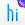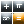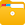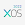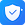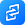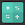# Calculator - Basic & Scientific Calculations

by Shalltry Group
10,000+ installs

Android application Calculator - Basic & Scientific Calculations developed by is listed under category Tools. According to Google Play Calculator - Basic & Scientific Calculations achieved more than 10,000 installs. Calculator - Basic & Scientific Calculations currently has 858 ratings with average rating value of 3.07. The current percentage of ratings achieved in last 30 days is 2.99%, percentage of ratings achieved in last 60 days is 5.74%. Calculator - Basic & Scientific Calculations has the current market position #132547 by number of ratings. A sample of the market history data for Calculator - Basic & Scientific Calculations can be found below. Last update on 2022-05-18.

Title: Calculator - Basic & Scientific Calculations Shalltry Group Tools Free Android
Total ratings: 858 2.99% 5.74% 3.07
Installs (achieved): 10,000+ 12,170
 5 star ratings: 411 34 0 34 377

2021-11-16: Android application Calculator - Basic & Scientific Calculations achieved 10,000 installs. Android application Calculator - Basic & Scientific Calculations achieved 5,000 installs. Android application Calculator - Basic & Scientific Calculations achieved 1,000 installs. Android application Calculator - Basic & Scientific Calculations achieved 500 installs.

Total number of ratings
Total number of active users rated for Calculator - Basic & Scientific Calculations.

Total number of installs (*estimated)
Estimation of total number of installs on Google Play. Approximated from number of ratings and install bounds achieved on Google Play.

Average rating
Average rating value on Google Play. Given by active users of the application.